# Declaration statements in C#

#### Statements:

Statements are the actions of the program which are expressed in Common methods, variables and values to execute the program.

Declaring a statement:

A declaration statement declares a new variable or constant with an expression, It assings a value for variable and assignment for constant.  A declaration statement encloses in a semicolon " " .

Example program to declare a statement:

```using System;
using System.Collections.Generic;
using System.Linq;
using System.Text;

class Program
{
static void Main()
{

int a = 1, b = 2, c = 5;
Console.WriteLine("{0} {1} {2}", a, b, c);

}
}
```

In the above code snippet we have declared how to declare the statement. In this program we have taken three int values a, b, c . We are defining a value as 1, b value as 2 and c value as 5. we have given console.writeline("{0} {1} {2}",a, b, c). which prints the output values as 1, 2 ,5.

output: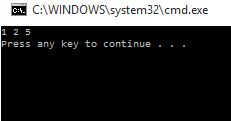#### Expression statement:

An expression represents a single data item which consists of single entity such as constant or variable. An expression can be followed by a semicolon. The execution of such statement causes an expression to be evaluated for example a=5

Example program on expression statement in c#:

```using System;
using System.Collections.Generic;
using System.Linq;
using System.Text;
class Program
{
static void Main()
{
int x, y;
x = 2 * 9;
x++;
y = Math.Min(x, 20);
Console.WriteLine(y);

x--;
y = Math.Max(x, 25);
Console.WriteLine(y);
}
}
```

In the above code snippet we have defined the expression, increment and decrement statement. we declared int values as x and y, we have assigned x value as 2*9=18.

In the next step we are incrementing (x++), Which Increments "x" with value 1, We are giving the condition y=Math.Min, which finds the minimum value as the "x" value i:e, 19 and "x" value (19) is the minimum value in the condition y=Math.Min(x,20) so it prints the minimum value as 19, We are calling method call expression which calls the y value as 19.

In the next step we are decrementing with (x--) and the x value is 19 and it is decremented with -1 it prints the value as 18, In this statement we are giving y=Math.Max (x,25), it prints the maximum value as 25. and in the method call expression it calls the y value as 25.

output: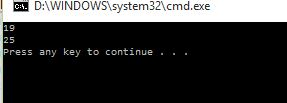#### Selection statements:

Selection statements are the number of possible statements which are used for the execution of the program. It causes the program control to be transferred to a specific flow based upon the certain condition is true or not.

if statement:

If statement is used to check whether the condition is true or false. An if statement is a programming conditional statement that, If proved true, performs a function or displays information.It is not specific to any particular programming language.

Example program on if statement:

```using System;
using System.Collections.Generic;
using System.Linq;
using System.Text;

class Program
{
static void Main()
{
int value = 20 * 2;
if (value == 40)
{
Console.WriteLine(true);
}
}
}
```

In the code snippetwe have defined the if statement. we have taken class as program, In the main function we are checking the if condition. We have given int value as `20*2` and in the next line we are checking the if condition `If (value==40)` . If the value is equal to 40 it prints the value as True

output:if Else statement:

If else statement is defined as two conditions if and else. It checks the two conditions if and else. When the if condition is true it executes and prints the value of if condition, When the if condition is false it executes in to the else condition and prints the value of else condition.

Example program on if else statement:

```using System;
using System.Collections.Generic;
using System.Linq;
using System.Text;
class Program
{
static void Main(string[] args)
{
int i;
Console.Write("Enter a Number : ");
if (i % 2 == 0)
{
Console.Write("Entered Number is an Even Number");
}
else
{
Console.Write("Entered Number is an Odd Number");
}
}
}
```

In the above code snippet we are checking the if else condition. We have taken class as program and in the main function we have taken int value as `i`, The console.Writeline prints the value as enter a number and `i=int.parse(console.ReadLine)`. The int.parse is used to convert the int value to low level type as int type and reads the i value. In the nextline we are checking the condition `if (i%2==0)` .  If the given i value is equal to zero it executes the if block and prints the value as Even number, If the given value is not equal to zero it executes the else block and prints the value as odd number.

outputSwitch statement:

Switch statement consists of more sections ,each section consists of case labels. switch statements results cleaner code than multiple if statements. Switch statement is a control statement that executes a set of logic functions. It is used to change the control of flow of program.

Example program on switch statement:

```using System;
using System.Collections.Generic;
using System.Linq;
using System.Text;

class Program
{
static void Main()
{
string value = "techfunda";
switch (value)
{
case "techfunda":
Console.WriteLine("techfunda");
break;
case "farmingfunda":
Console.WriteLine("farmingfunda");
break;
case "kidsfunda":
Console.WriteLine("kidsfunda");
break;
}
}
}
```

In the above code snippet we are checking the switch statement. We defined class as program and in the main function we have taken string value as Techfunda. In the nextline we are checking the switch statement as switch(value) , We are giving the case statements as `case Techfunda` and the console.Writeline prints the techfunda, The break statement is executed, In` case farmingfunda` the console.Writeline prints as farming funda and the break statement is executed  in `case kidsfunda` the console.writeline prints the value as kidsfunda and the break statement is executed, but the output is printed as techfunda because we have given string value as techfunda.

outputJump statements:

Jump statements are used to branch the operations.They are different types of jump statements

• Break statement
• Continue statement
• Return statements
• Throw statement
• GoTo statement

Break statement:

Break statement is one of the control statements used in certain parts of a program. When the break statement is encountered inside a loop, the loop is immediately terminated and program control resumes at the next statement following the loop.

Example program on break statement

```using System;
using System.Collections.Generic;
using System.Linq;
using System.Text;
public class Example
{
static void Main()
{
for (int i = 1; i <= 10; i++)
{
if (i == 5)
{
break;
}
Console.WriteLine(i);
}
Console.WriteLine("Next statement placed after loop");
}
}
```

In the above code snippet we have declared break statement. In this break statement we have used for statement for checking the condition if i value is less than 10 or equal to 10, we have declared the i value as 1 and if the value of i is less than 10 means it increments with `i++` condition and again the value checks with 10, we have given the the condition if `i==5` we used break statement if the i value is equal to 5 break statement executes and stop executing the output values.

outputContinue statement:

The continue statement passes control to the next iteration of the enclosing statement in which it appears. The continue statement skips the loop and increment or decrement it by one.

Example program on continue statement:

```using System;
using System.Collections.Generic;
using System.Linq;
using System.Text;
class program
{
static void Main()
{
for (int i = 1; i <= 20; i++)
{
if (i < 6)
{
continue;
}
Console.WriteLine(i);
}
Console.WriteLine("Press any key to exit.");
}
}
```

In the above code snippet we have defined continue statement.we have used for condition for checking the i value if the value of i is equal or less than 20. if the value is less than 20 means it increments with the condition `i++` we are checking with the condition if `(i<6)`  then we have given the continue statement, the values starts executing from the value 6. and prints the values of i.

output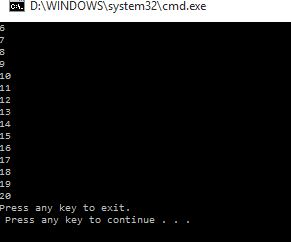Return statement;

The return statement terminates execution of the method in which it appears and returns control to the calling method. It can also return an optional value.

Example program on return statement:

```using System;
using System.Collections.Generic;
using System.Linq;
using System.Text;
class Return
{
static double CalculateArea(int r)
{
double area = r * r * Math.PI;
return area;
}

static void Main()
{
Console.WriteLine("The area is {0:0.00}", result);

Console.WriteLine("Press any key to exit.");
}
}
```

In the above code snippet we have defined the return statement. We have taken class as return and we have taken function as `double calculate Area().` In this function we are assigning the `area` value by` r*r*MATH.PI` and return area, In the main function of the program we are defining the int r as 8 and given the double result  as function calculate Area(radius). and we have given the console.writeline as area{0:0:00} which prints the area value  and prints the output value  as 201

output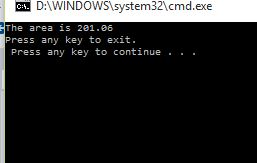Throw statement:

The throw statement is used to signal the occurrence of an situation (exception) during the program execution.

Example program on Throw statement

```using System;
using System.Collections.Generic;
using System.Linq;
using System.Text;
public class Throw
{

static int GetNumber(int index)
{
int[] nums = { 300, 600, 900 };
if (index > nums.Length)
{
throw new IndexOutOfRangeException();
}
return nums[index];

}
static void Main()
{
int result = GetNumber(3);

}
}
```

In the above code sniipet we have defined the throw statement. We have taken class as Throw we have taken function as `int GetNumber(int index)`, In this function we have taken int numbers as {300, 600, 900} and we have taken if condition` if(index>nums.Length).` In this if function we have throw new IndexOutOf RangeException() and return nums[index], In this main function we have int result as getnumber(3). which prints the output as Throw new exception.

output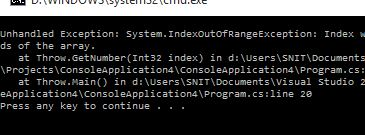GoTo statement:

The Goto statement transfers the program control directly to a labeled statement. The common use of goto is to transfer control to a specific switch-case label or the default label in a switch statement.

Example program on goto statement

```using System;
using System.Collections.Generic;
using System.Linq;
using System.Text;
class Switch
{
static void Main()
{
Console.WriteLine("pizza sizes: 1=Small 2=Medium 3=Large");
int n = int.Parse(s);
int cost = 0;
switch (n)
{
case 1:
cost += 25;
break;
case 2:
cost += 25;
goto case 1;
case 3:
cost += 50;
goto case 1;
default:
Console.WriteLine("Invalid selection.");
break;
}
if (cost != 0)
{
}

Console.WriteLine("Press any key to exit.");
}
}
```

In the above code snippet we have defined the Goto statement using switch case statement. We have taken class as switch and in the main function we have declared console.writeline to print the enter small ,medium and large and in the nextline we have given the console.write to select the values` int n=int.parse(s)` which prints the n value. In the switch case we have given the cases as 1 =cost=25 break, it is defined as 25cents  and case2 as +=25 gotocase1, It is defined as 25 and goto case1 which increments with 25 and case3 as +=50, goto case1, It is defined as 50 and increments with case1 condition. If the value not dequal to zero it prints the value as invalid and thanks for your business.

output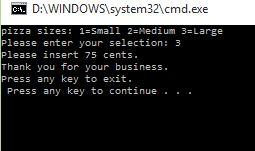Iteration statements:

An iteration statement, in  C#, is defined as a block of code that returns an ordered sequence of values of a collection or arrays. They are the sequence of statements which executes rapidly with while, do while, for, for each statements.

They are different iteration statements.

• while statement
• do while statement
• for statement
• for each statement

While statement:

While statements are used to repeatedly execute a body of code. The expression is tested before the body of the code.The while statement, in C#, is an iteration statement that allows for the execution of an statement conditionally for zero or more times.

Example program on while statement:

```using System;
using System.Collections.Generic;
using System.Linq;
using System.Text;
class Program
{
static void Main()
{
int i = 5;
while (i < 20)
{
i++;
Console.Write("TECHFUNDA ");
Console.WriteLine(i);
}
}
}
```

In the above code snippet we have declared while loop, we have declared int value as 5 and we used while condition for checking` (i<20)`. the i value 5 is less than 20 and the condition `i++`` ` increments the value 5 to 6 and we have printedtechfunda in console.writeline which prints the value as techfunda 6 and this repeats until the i value is equal to 20.

output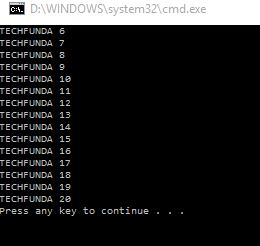Do While statement:

Do while statement comes before the condition. A do while loop is a control flow statement that executes a block of code at least once, and then repeatedly executes the block.

Example program on Do While statement

```using System;
using System.Collections.Generic;
using System.Linq;
using System.Text;
class Program
{
static void Main(string[] args)
{
int a = 2;
do
{
Console.WriteLine("value of a: {0}", a);
a = a + 1;
}
while (a < 8);
}
}
```

In the above code snippet we are declaring the dowhile condition,  We have taken int value a as 2 we are checking with the condition do. we have given console.writeline  and it prints the value of a and it increments the value of a with 1 and in the next line we have the condition `while (i<8)` where it checks the value  of  a if  value is lessthan 8 it increments the value of a until it is equal to 8.

outputFor statement:

For loop is like while loop which consists of three conditions in the statement the initilisation, condition, iteration. For loop is used to execute the code repeatedly.

Example program on for statement:

```using System;
using System.Collections.Generic;
using System.Linq;
using System.Text;

class Program
{
static void Main()
{
for (int i = 0; i < 10; i++)
{
Console.WriteLine(i);
}
}
}
```

In the above code snippet we have declared the for loop statement, we are checking three conditions initilisation,conditioniteration`i=0, i<10, i++`, If the i value is 0 then the value of i is less than 10, It increments until the value of i is equal to the 10 value

outputFor each loop statement:

For each loop statement is used to iterate the elements in a sequence. It does not include initilization, increment and termination.

Example program on for each loop statement:

```using System;
using System.Collections.Generic;
using System.Linq;
using System.Text;
class Program
{
static void Main(string[] args)
{
string[] arr = new string;

arr = "TechFunda";
arr = "dotnetfunda";
arr = "itfunda";
arr = "kidsfunda";
arr = "farmingfunda";
arr = "fundoo video";

foreach (string name in arr)
{
Console.WriteLine("Hello " + name);
}
}
}

```

In the above code snippet we have defined the foreach loop statement. We have declared class as program and in the ,main function we have defined `string[] arr =new string ` and we have declared the array values as `arr= "TechFunda" , arr="dotnetfunda", arr="itfunda", arr="kidsfunda", arr="farmingfunda", arr="fundoovideo"` and in the nextline we have given the condition `foreach(string name in arr)` and in the console.writeline("Hello " +name); which prints the names with Hello and the console.Readline() as which reads the names as output.

output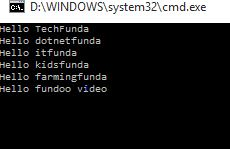Views: 12980 | Post Order: 13